# CAIIB ABFM Module C Unit 2 : Discounted Cash Flow Valuation

## CAIIB Paper 3 ABFM Module C Unit 2 : Discounted Cash Flow Valuation (New Syllabus)

IIBF has released the New Syllabus Exam Pattern for CAIIB Exam 2023. Following the format of the current exam, CAIIB 2023 will have now four papers. The CAIIB Paper 3 (ADVANCED CONCEPTS OF FINANCIAL MANAGEMENT) includes an important topic called “Discounted Cash Flow Valuation”. Every candidate who are appearing for the CAIIB Certification Examination 2023 must understand each unit included in the syllabus.

In this article, we are going to cover all the necessary details of CAIIB Paper 3 (ABFM) Module C (Valuation, Mergers & Acquisitions) Unit 2 : Discounted Cash Flow Valuation, Aspirants must go through this article to better understand the topic, Discounted Cash Flow Valuation and practice using our Online Mock Test Series to strengthen their knowledge of Discounted Cash Flow Valuation. Unit 2 : Discounted Cash Flow Valuation

### Estimating Inputs

For applying the DCF method of valuing a firm or any other asset, we need the following inputs:

• The predicted cash flows in future
• The discount rate that is suitable for the given level of risk associated with these cash flows
• The estimated cash flow growth rate and
• The estimated pattern of growth.

#### Expected Cash Flows

• The dividend is the only source of cash flow that an equity investor receives from a publicly traded company, financial models that use dividends as cash flows are referred to as dividend discount models.
• A broader definition of cash flows to equity would be the cash flows that are left over after the cash flow claims of non-equity investors in the firm have been met (interest and principal payments to debt holders and preferred dividends), as well as after enough of these cash flows have been reinvested into the firm to sustain the projected growth in cash flows. This concept is known as the free cash flow to equity (FCFE), and the types of models that make use of this concept, are referred to as FCFE discount models.
• The total cash flow to all claim holders in the company is what is referred to as the cash flow to the firm. One technique to calculate this cash flow is to add the free cash flows to equity to the cash flows to lenders (debt) and preferred stockholders. The models that make use of these cash flows are referred to as FCFF models, and this cash flow is referred to as the free cash flow to the firm (FCFF).

#### Discount Rates

• Discounting determines the present value of future cash flows by using a rate that is the cost of capital that most accurately reflects the risk and timing of the cash flows. This rate is called the discount rate.
• Cash flows with a higher level of risk should have higher discount rates. There are two different perspectives on risk. The first type of risk is known as default risk, When looking at debt, the rate that reflects the possibility of default is referred to as the cost of debt.
• The second method to look at risk is to consider how it relates to the difference between actual returns and expected returns. The actual returns on an investment with a high level of risk, may be considerably different from the predicted returns; the bigger the deviation, the higher the level of risk.
• The cost of capital can be determined by taking the average of the cost of equity, as well as the cost of borrowing money after taxes, which is determined by the default risk, and then weighting the average by the proportions that are used for each type of funding.
• The discount rates, that are applied in discounted cash flow valuations, ought to be reflective of the riskiness of the cash flows being valued. To be more specific, the cost of debt needs to include a default premium or spread to account for the risk of the debt going into default, and the cost of stock needs to include a risk premium to account for the risk of equity.

#### Estimating future Growth

• We need estimates of the predicted growth rate for revenues and the expenses, in order to make projections about future cash flows. The rate of inflation, that is anticipated, is an essential component in the process of predicting the growth rate.
• There are three different methods that can be used to estimate growth. One strategy is to investigate the past of a company and employ the historical growth rate that was reported by that company.
• The second method is to get estimates of growth from sources that have a greater level of expertise. For some analysts, this means using the estimates provided by a company’s management, while for others, it takes the form of employing the consensus estimates of growth produced by others who follow the firm.
• The third method is to conduct a survey. The surveys are used to collect information on the expectations that investors have for predicted growth.

#### Estimating Growth Patterns

When valuing a company in general, there are three main approaches, that may be taken, for the growth pattern:

• We can assume that the company is already in stable growth
• We can assume a time of consistent high growth and subsequently lower growth rate to stable growth (two-stage growth); or
• We can provide for an interim period to get to sustained growth (three-stage or n-stage models).

The rate of expansion of a company over the most recent time period allows us to divide businesses into three distinct categories.

• Companies considered to have stable growth report earnings and revenue growth that is equal to or lower than the nominal growth rate of the economy in which they operate.
• Companies are considered to have moderate growth if their earnings and revenue grow at a rate that is just slightly faster than the economy’s nominal growth rate.

The earnings and sales of high-growth companies expand at a rate that is significantly faster than the nominal growth rate of the economy as a whole.

The steady state models that assume constant growth provide accurate estimates of value for businesses that are growing at a stable rate. The two-stage discounted cash flow model should give sufficient flexibility in terms of capturing changes in the underlying features of the firm for businesses that are developing at a rate that is considered to be moderate. It is possible that a model with three stages or even more stages may be required to reflect the longer transitions to steady growth that are characteristic of high-growth companies.

### Approaches To Discounted Cash Flow Models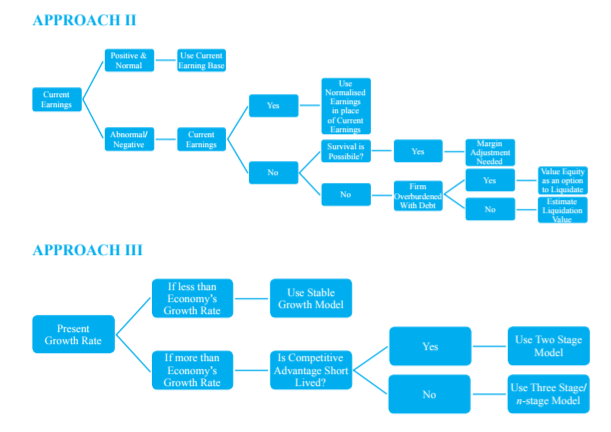### Various Discounted Cash Flow Models

• Enterprise DCF Model: The enterprise DCF model applies a discount rate equal to the weighted average cost of capital to the free cash flow to the firm (FCFF).
• Equity DCF Model: It can be implemented in two different ways: the dividend discount model and the free cash flow to equity model. The dividend discount model takes into account the cost of equity when calculating a discount on the expected dividend stream. The free cash flow to equity model also takes into account the cost of equity when calculating a discount on the free cash flow to equity.
• Adjusted Present Value (APV) Model: The APV model discounts the unlevered equity cash flow (which is the same as the free cash flow to the firm) at the unlevered cost of equity (the cost of equity assuming the firm has no leverage) and adds to it the discounted value of the interest tax shield on debt. This results in an estimate of the present value of the unlevered equity cash flow.
• The Economic Profit Model: In the economic profit model, the economic profit stream is discounted using the weighted average cost of capital, and then the currently invested capital is added to the resulting value.

### Dividend Discount Model

The Dividend Discount Model is a quantitative method of valuing a company’s equity shares price based on the assumption that the fair price of share equals the present value of the company’s future dividends.

The various dividend discount models used for valuation are as under:

• Constant Growth Model
• Zero Growth Model
• Two Stage Model
• H Model
• Three Stage Model

#### Constant Growth Model

One of the most common assumptions used by dividend discount models is that the dividend paid out per share would increase at a rate that is fixed (g). According to this hypothesis, the value of a share is computed as follows: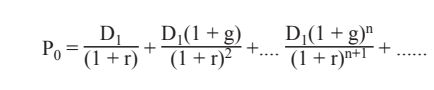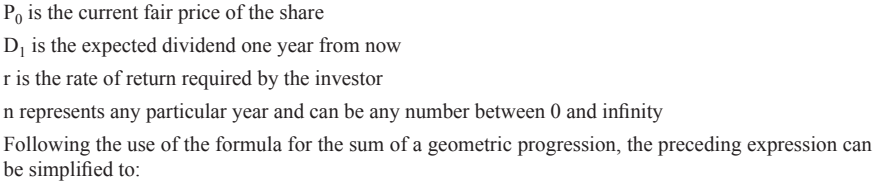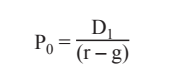Illustration:

XYZ Ltd. is expected to pay a dividend of Rs. 3 per share one year from now. The dividend payments are expected to grow at 5% p.a. If an investor needs 12% rate of return on his investment, what price of the share will be considered to be fair, by him.

Solution:

Applying the formula, given above,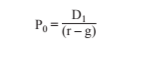We get Po = 3/ (0.12 – 0.05) = 3/ 0.07 = Rs. 42.86

The vast majority of stock valuation models are founded on the idea that dividends will increase over the course of time.

#### Zero Growth Model

In the event that we make the assumption that the dividend per share stays the same from year to year at a value of D, the formula will look like this:

Po= D/r

Illustration:

XYZ Ltd. is expected to pay a dividend of Rs. 3 per share one year from now. The dividend payments are expected to remain constant at Rs. 3 per share. If an investor needs 12% rate of return on his investment, what price of the share will be considered to be fair, by him.

Solution:

Applying the formula for fair value,#### Two Stage Model

• It is based on the assumption of two primary stages of dividend growth: that the exceptional growth—whether positive or negative—will last for a set number of years, after which the usual growth rate will take over and continue indefinitely.
• In the event where the dividends increase at a rate that is proportional to the rate of growth, the market price of the equity share will be: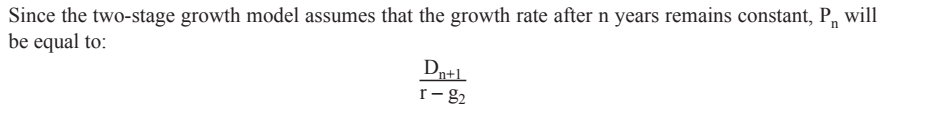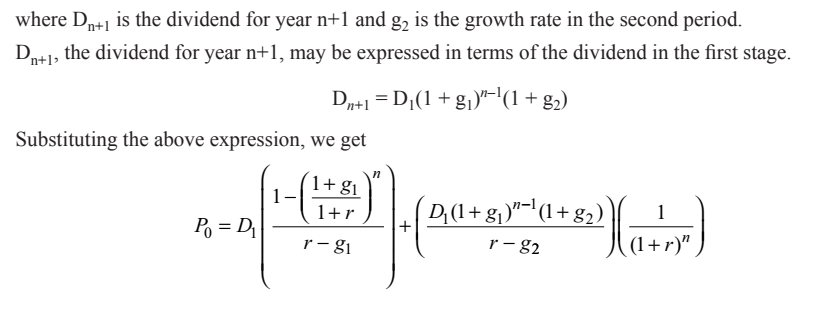Illustration:

The current dividend on an equity share of PML Private Limited is Rs. 5. PML is expected to enjoy an above-normal growth rate of 25 percent for a period of 6 years. Thereafter, the growth rate will fall and stabilise at 15 percent. Equity investors require a return of 20 percent. What is the intrinsic value of the equity share of PML?

Solution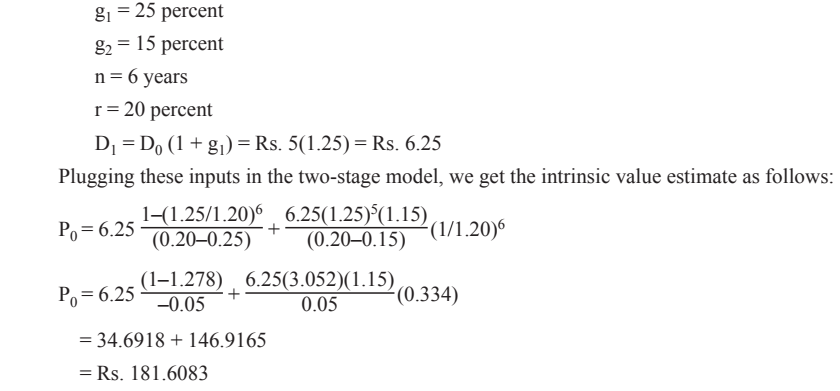#### H Model

• The H model, as opposed to the traditional two-stage model, postulates that the exceptional growth rate in the beginning stage does not remain constant but rather decreases linearly over time until it achieves a stable rate in the steady stage.
• The H model, which was developed by Fuller and Hsia, makes the assumption that the earnings growth rate starts off at a high initial rate ( ), and then decreases at a linear rate over the course of 2H years to a stable growth rate (), which is maintained forever. This model also assumes that the earnings growth rate will remain stable forever, after the stability has been achieved. It operates under the presumption that the dividend payment rate and the cost of equity will not change over the course of time and will not be affected by the fluctuating growth rates.Illustration:

The equity shares of ABC Private Limited presently generate a dividend payment of Rupee 1.00 every year. The growth rate as of right now is thirty percent. On the other hand, this will decrease in a linear fashion over the course of ten years, and then it will level off at ten percent. What is the company’s intrinsic worth per share, assuming that investors require a return of 15% on their investment?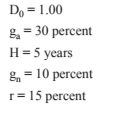Putting the above inputs in the H-model, we get the estimated intrinsic value as follows:The H model is more realistic than the two-stage model, which predicts that the growth rate would suddenly slow down after a given amount of time. Instead, the H model predicts that the growth rate will gradually slow down over time. On the other hand, presuming that the dividend pay-out rate will remain unchanged during all stages of growth appears to be an unrealistic expectation. Because of this, the model cannot be used by any company that pays out zero or very little dividends at the present time.  The applicability of the model is severely restricted due to the requirement that it exhibit both rapid development and substantial pay-outs.

#### Three Stage Model

Combining the two-stage model with the H model resulted in the creation of the three-stage growth model.  It presupposes that there will be an initial time of stable high growth, a second period of growth that will decrease in a linear fashion, and a third era of steady low growth that will continue on indefinitely.